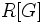# Galois module

(Redirected from Galois representation)
Let$L/K$ be a Galois etension of fields. Then, a Galois module for this extension, relative to a ring$R$, is defined as a module over the group ring$R[G]$, where$G$ is the Galois group of$L/K$.
In the case where$R=k$, a field, this is equivalent to a linear representation of$G$ over$k$. Thus, we also use the term Galois representation.